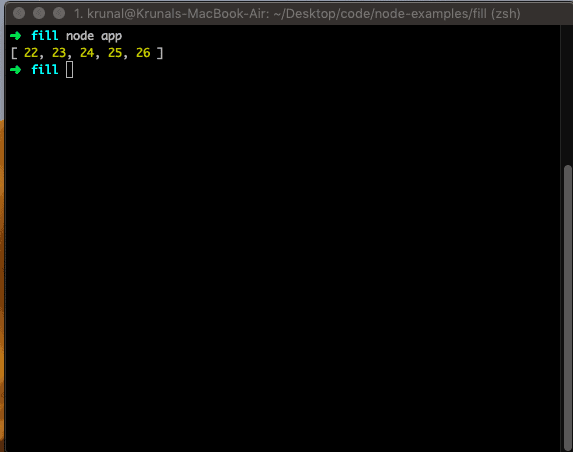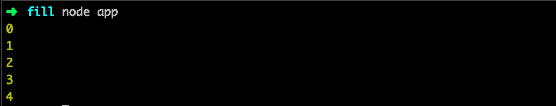# JavaScript Array forEach() Method

The forEach() is a built-in JavaScript method of arrays that allows you to loop over the elements of an array and execute a callback function for each element.

### Syntax

``array.forEach(function(currentValue, index, arr), thisValue)``

### Parameters

The currentValue is the current item in an array that is iterating.

The index is an optional parameter, which is the current element index of an array.

The arr is an optional parameter, an array object to which the current element belongs.

The thisValue is an optional parameter that is the value to be passed to the function to be used as its “this” value.

### Example

An Array.forEach() function calls the provided function once for each array element.

The provided function may perform any operation on the elements of the given array.

Let us create a folder, and inside that, create a file called app.js and add the following code.

``````var arr = [1, 2, 3, 4, 5];
var newArray = [];

arr.forEach(function(item) {
newArray.push(item + 21);
});

console.log(newArray);``````

In the above example, we use the forEach function to add 21 to every array item. So the newly created element looks like this.

OutputWe can write the above function in the ES6 style.

``````let arr = [1, 2, 3, 4, 5];
let newArray = [];

arr.forEach(item => newArray.push(item + 21));

console.log(newArray);``````

The forEach() executes the callback function once for each array element; unlike map() or reduce(), it always returns the value undefined, and it is not chainable. So we can use it to execute at the end of a chain.

## How to access the array index in the forEach() method

JavaScript’s forEach() function takes a callback as a parameter and calls that callback for each array element. The callback function can take up to three arguments: the current element being processed in the array, its index, and the array itself.

``````let arr = [1, 2, 3, 4, 5];
let newArray = [];

arr.forEach((item, i) => console.log(i));``````

OutputThere is no way to break the forEach() function other than throwing an exception. If you need such behavior for your code, then the forEach method is not an option here.

## How to Break forEach()

You cannot directly break out of a forEach() loop. However, there are some alternatives you can use:

1. Use array.every() instead of forEach(). The every() function behaves exactly like forEach(), except it stops iterating if the callback returns false.
2. Filter out the values you want to skip.

## Difference between forEach() and for in loop

The forEach() method is used to loop through arrays. It passes a callback function for each element of an array together with the current value (required), index (optional), and array (optional). On the other hand, a for…in loop iterates over the enumerable properties of an object. The order of iteration is not guaranteed.

That’s it.

### 1 thought on “JavaScript Array forEach() Method”

1.thanks for the post